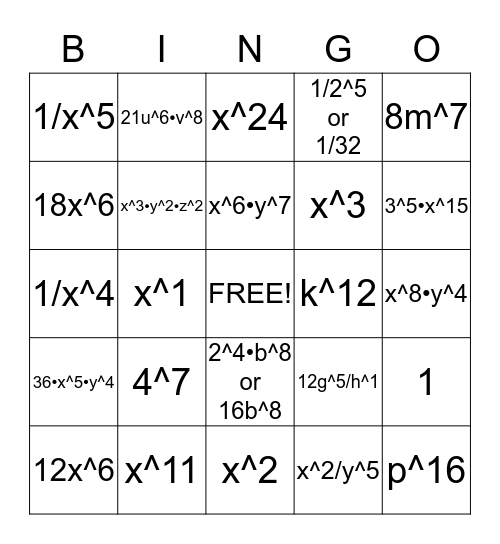# Exponent ReviewThis bingo card has a free space and 24 words: 4^7, x^11, x^6•y^7, 12x^6, 8m^7, 1/x^5, 18x^6, 21u^6•v^8, k^12, 2^4•b^8 or 16b^8, x^3, 1/x^4, 12g^5/h^1, 1, x^3•y^2•z^2, x^2/y^5, x^8•y^4, x^24, 3^5•x^15, x^1, p^16, 36•x^5•y^4, 1/2^5 or 1/32 and x^2.

## Play Online